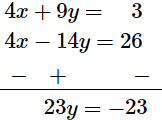Deepak Scored 45->99%ile with Bounce Back Crack Course. You can do it too!

# Find the real values of x and y, if

Question:

Find the real values of x and y, if

(i) $(x+i y)(2-3 i)=4+i$

(ii) $(3 x-2 i y)(2+i)^{2}=10(1+i)$

(iii) $\frac{(1+i) x-2 i}{3+i}+\frac{(2-3 i) y+i}{3-i}$

(iv) $(1+i)(x+i y)=2-5 i$

Solution:

(i) $(x+i y)(2-3 i)=4+i$

$2 x-3 i x+2 i y-3 i^{2} y=4+i$

$2 x+3 y+i(-3 x+2 y)=4+i$

Comparing both the sides:

$2 x+3 y=4 \quad \ldots .(1)$

$-3 x+2 y=1 \quad \ldots .(2)$

Multiplying equation (1) by 3 and equation (2) by 2 :

$6 x+9 y=12 \quad \ldots(3)$

$-6 x+4 y=2 \quad \ldots(4)$

Adding equations$(3)$ and $(4)$ :

$13 y=14$

$y=\frac{14}{13}$

Substituting the value of $y$ in equation $(1)$ :

$2 x+3 \times \frac{14}{13}=4$

$\Rightarrow 2 x=4-\frac{42}{13}$

$\Rightarrow 2 x=\frac{10}{13}$

$\Rightarrow x=\frac{5}{13}$

$\therefore x=\frac{5}{13}$ and $y=\frac{14}{13}$

(ii) $(3 x-2 i y)(2+i)^{2}=10(1+i)$

$\Rightarrow(3 x-2 i y)\left(4+i^{2}+4 i\right)=10(1+i)$

$\Rightarrow(3 x-2 i y)(3+4 i)=10(1+i)$

$\Rightarrow 9 x+12 x i-6 i y-8 i^{2} y=10+10 i$

$\Rightarrow 9 x+8 y+i(12 x-6 y)=10+10 i$

Comparing both the sides :

$9 x+8 y=10 \quad \ldots .(1)$

$12 x-6 y=10$

or, $6 x-3 y=5 \quad \ldots(2)$

Multiplying equation $(1)$ by 3 and equation $(2)$ by 8 ,

$27 x+24 y=30$      ...(3)

$48 x-24 y=40$    .....(4)

Adding equations $(3)$ and $(4)$ :

$75 x=70$

$\therefore x=\frac{14}{15}$

Substituting the value of $x$ in equation $(1):$

$9 \times \frac{14}{15}+8 y=10$

$\Rightarrow \frac{126}{15}+8 y=10$

$\Rightarrow 8 y=10-\frac{126}{15}$

$\Rightarrow 8 y=\frac{24}{15}$

$\Rightarrow y=\frac{1}{5}$

(iii) $\frac{(1+i) x-2 i}{3+i}+\frac{(2-3 i) y+i}{3-i}=i$

$\Rightarrow \frac{(1+i)(3-i) x-2 i(3-i)+(2-3 i)(3+i) y+i(3+i)}{(3+i)(3-i)}=i$

$\Rightarrow \frac{3 x-i x+3 i x-i^{2} x-6 i+2 i^{2}+6 y+2 i y-9 i y-3 i^{2} y+3 i+i^{2}}{9-i^{2}}=i$

$\Rightarrow \frac{4 x+2 i x-3 i+9 y-7 i y-3}{10}=i$

$\Rightarrow(4 x+9 y-3)+i(2 x-3-7 y)=10 i$

Comparing both the sides :

$4 x+9 y-3=0$

$\Rightarrow 4 x+9 y=3$    ...(1)

$2 x-3-7 y=10$

$\Rightarrow 2 x-7 y=13$   ,....(2)

Multiplying equation $(2)$ by 2 :

$4 x-14 y=26 \quad \ldots(3)$

Subtracting equation $(3)$ from $(1)$ :$\therefore y=-1$

Substituting the value of $y$ in equation $(1):$

$4 x-9=3$

$\Rightarrow 4 x=12$

$\Rightarrow x=3$

$\therefore x=3$ and $y=-1$

(iv) $(1+i)(x+i y)=2-5 i$

$\Rightarrow x+i y+i x+i^{2} y=2-5 i$

$\Rightarrow x+i y+i x-y=2-5 i$

$\Rightarrow(x-y)+i(y+x)=2-5 i$

Comparing both the sides,

$x-y=2$    ....(1)

$x+y=-5$      ....(2)

Adding equations $(1)$ and $(2)$,

$2 x=-3$

$\Rightarrow x=\frac{-3}{2}$

Substituting the value of $x$ in equation $(1)$,

$\frac{-3}{2}-y=2$

$\Rightarrow y=\frac{-3}{2}-2$

$\Rightarrow y=\frac{-7}{2}$

$\therefore x=\frac{-3}{2}$ and $y=\frac{-7}{2}$